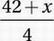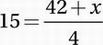# SAT Math Multiple Choice Question 678: Answer and Explanation

### Test Information

Question: 678

3. A certain function takes an input value and transforms it into an output value according to the following three-step procedure:

Step 1: Multiply the input value by 6.

Step 2: Add x to this result.

Step 3: Divide this result by 4.

If an input of 7 to this function yields an output of 15, what is the value of x?

• A. 12
• B. 16
• C. 18
• D. 24

Explanation:

C

Let the input number be 7.

Step 1: Multiply the input value by 6:

42

Step 2: Add x to that result:

42 + x

Step 3: Divide this result by 4:This must yield an output of 15:Multiply by 4:

60 = 42 + x

Subtract 42:

18 = x